# Excel time format & how to use NOW and TIME functions to insert time

In this tutorial, you will learn the specificities of Excel time format as well as how to add a timestamp using shortcuts or insert an auto updatable time with the NOW function. You will also learn how to apply special Excel time functions to get hours, minutes or seconds from a time stamp.

Microsoft Excel has a number of helpful time features and knowing them a little in depth can save you a lot of time. Using special functions, you can insert the current date and time anywhere in a worksheet, convert time to a decimal number, sum different time units or calculate the elapsed time.

To be able to leverage powerful Excel time functions, it helps to know how Microsoft Excel stores times. So, before digging deeper into the formulas, let's invest a couple of minutes in learning the basics of the Excel time format.

## Excel time format

If you have been following our Excel Dates tutorial, you know that Microsoft Excel stores dates as sequential numbers beginning with January 1, 1900, which is stored as number 1. Because Excel treats time as a portion of a day, times are stored as decimal fractions.

In Excel's internal system:

• 00:00:00 is stored as 0.0
• 23:59:59 is stored as 0.99999
• 06:00 AM is 0.25
• 12:00 PM is 0.5

When both a date and time are entered in a cell, they are stored as a decimal number comprised of an integer representing the date and a decimal portion representing the time. For example, 1 June 2015 9:30:00 AM is stored as 42156.3958333333.

### How to get a decimal representing time in Excel

A quick way to pick a decimal number representing a certain time is to use the Format Cells dialog.

Simply select a cell containing the time and press Ctrl + 1 to open the Format Cells dialog box. On the Number tab, select General under Category, and you will see the decimal in the Sample box.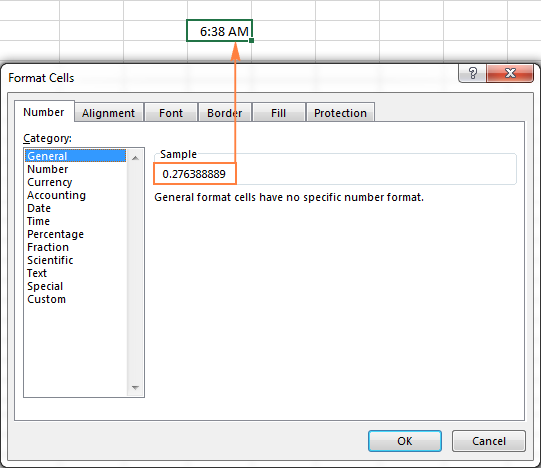Now, you can write down that number and click Cancel to close the window. Or, you can click the OK button and have the time replaced with a corresponding decimal number in the cell. In fact, you can think of it as the fastest, easiest and formula-free way to convert time to decimal in Excel. In the next part of our Excel Time tutorial, we'll take a closer look at special time functions and calculations to convert time to hours, minutes or seconds.

### How to apply or change the time format in Excel

Microsoft Excel is smart enough to recognize a time as you type and format the cell accordingly. For example, if you type 20:30, or 8:30 PM, or even 8:30 p in a cell, Excel will interpret this as a time and display either 20:30 or 8:30 PM, depending on your default time format.

If you want to format some numbers as times or apply a different time format to existing time values, you can do this by using the Format Cells dialog, as demonstrated below.

1. In an Excel sheet, select the cell(s) where you want to apply or change the time format.
2. Open the Format Cells dialog either by pressing Ctrl + 1 or by clicking the Dialog Box Launcher icon next to Number in the Number group, on the Home tab.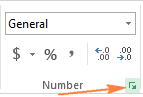3. On the Number tab, select Time from the Category list, and choose the desired time format from the Type list.
4. Click OK to apply the selected time format and close the dialog box.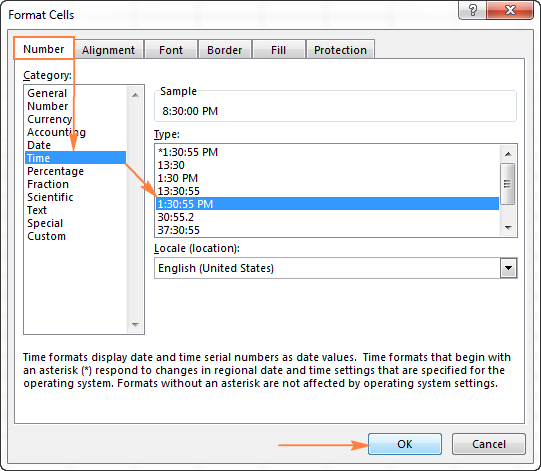### Creating a custom Excel time format

Though Microsoft Excel provides a handful of different time formats, you may want to create your own one that fits the best for a particular sheet. To do this, open the Format Cells dialog box, select Custom from the Category list and type the time format you want to apply in the Type box.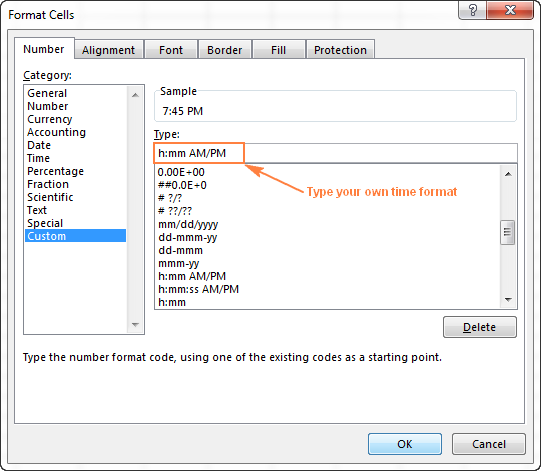The custom time format you've created will be in the Type list the next time you need it.

Tip. The easiest way to create a custom time format in Excel is to use one of the existing formats as a starting point. For this, click Time in the Category list, and select one of the predefined formats under Type. After that switch to Custom and make the changes to the format displayed in the Type box.

When creating a custom time format in Excel, you can use the following codes.

 Code Description Displays as h Hours without a leading zero 0-23 hh Hours with a leading zero 00-23 m Minutes without a leading zero 0-59 mm Minutes with a leading zero 00-59 s Seconds without a leading zero 0-59 ss Seconds with a leading zero 00-59 AM/PM Periods of the day (if omitted, 24-hour time format is used) AM or PM

Tip. To create custom formatting for dates and time, use various combinations of time codes and date codes.

The following table provides a few example of how your Excel time formats may look like:

 Format Displays as h:mm:ss AM/PM 1:30:00 PM h:mm 13:30 dddd, m/d/yy h:mm AM/PM Tuesday, 1/13/15 1:30 PM ddd, mmmm dd, yyyy hh:mm:ss Tue, January 13, 2015 13:30:00

#### Custom formats for time intervals over 24 hours

When you are calculating times in Excel, a cell containing the sum of time amounts may exceed 24 hours. To get Microsoft Excel to display times beyond 24 hours correctly, apply one of the following custom time formats.

 Format Displays as Explanation [h]:mm 41:30 41 hours and 30 minutes [h]:mm:ss 41:30:10 41 hours, 30 minutes and 10 seconds [h] "hours", mm "minutes", ss "seconds" 40 hours, 30 minutes, 10 seconds d h:mm:ss 1 17:30:10 1 day, 17 hours, 30 minutes and 10 seconds d "day" h:mm:ss 1 day 17:30:10 d "day," h "hours," m "minutes and" s "seconds" 1 day, 17 hours, 30 minutes and 10 seconds

#### Custom formats for negative time values

The custom time formats discussed above work for positive values only. If the result of your calculations is a negative number formatted as time (e.g. when you subtract a bigger time amount from a smaller one), the result will be displayed as #####. If you want to format negative time values differently, the following options are available to you:

• Display an empty cell for negative times. Type a semicolon at the end of the time format, for example [h]:mm;
• Display an error message . Type a semicolon at the end of the time format, and then type a message in quotation marks, e.g. [h]:mm;"Negative time"

Generally speaking, a semicolon acts as a delimiter to separate positive values' format from negative values' formatting.

If you want to display negative times as negative values, e.g. -10:30, the easiest way is to change the Excel's Date System to 1904 date system. To do this, click File > Options > Advanced, scroll down to When calculating this workbook section and check Use 1904 date system box.

### The default time format in Excel

When changing the time format in the Format Cells dialog, you may have noticed that one of the formats begins with an asterisk (*). This is the default time format in your Excel.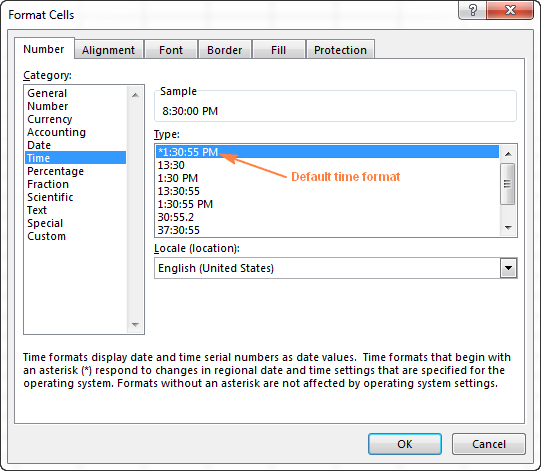To quickly apply the default Excel time format to the selected cell or a range of cells, click the drop-down arrow in the Number group, on the Home tab, and select Time.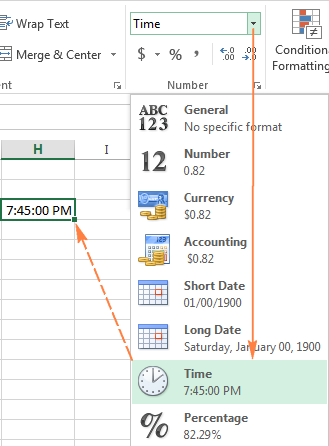To change the default time format, go to the Control Panel and click Region and Language. If in your Control panel opens in Category view, click Clock, Language, and Region > Region and Language > Change the date, time, or number format.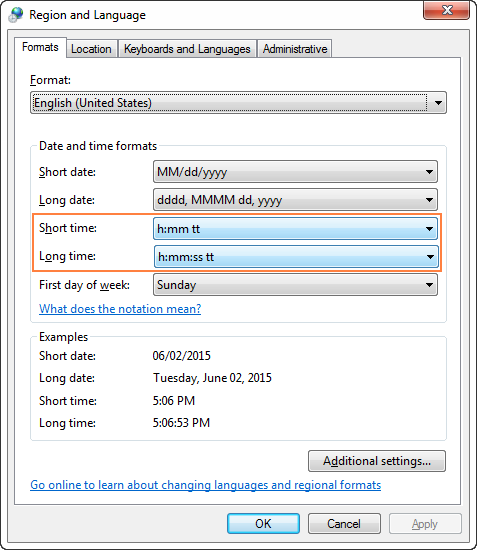Note. When creating a new Excel time format or modifying an existing one, please remember that regardless of how you've chosen to display time in a cell, Excel always internally stores times the same way - as decimal numbers.

## How to insert time and a timestamp in Excel

There are a numbers of ways to insert time in Excel, which one to use depends on whether you want a static timestamp or a dynamic value that updates automatically to reflect the current time.

### Add time stamp using shortcuts

If you are looking for a way to insert a timestamp in an Excel cell, i.e. a static value that won't automatically update whenever a workbook is recalculated, then use one of the following shortcuts:

• To insert the current time, press Ctrl + Shift + ;
• To enter the current date and time, press Ctrl + ; which inserts a date, then press the Space key, and then hit Ctrl + Shift + ; to insert the current time.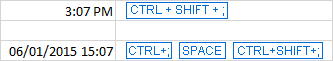### Add today's date and current time using NOW function

If you aim to insert the current date and time as a dynamic value that refreshes automatically, then use the Excel NOW function.

The formula is as simple as it can possibly be, no arguments are required:

`=NOW()`

When using the NOW function in Excel, there are a few things to keep in mind:

• The NOW function retrieves time from your computer's system clock.
• NOW is one of Excel's volatile functions that cause the cell with the formula to recalculate every time the worksheet is re-opened or recalculated.
• To force the Excel NOW function to update the returned value, press either Shift + F9 to recalculate the active worksheet or F9 to recalculate all open workbooks.
• To get the NOW function to automatically update at a specified time interval, add a VBA macro to your workbook, a few examples are available here.

### Insert current time as a dynamic value

If you'd rather insert only the current time in a cell without a date, you have the following choices:

1. Use the NOW fucntion, and then apply the time format to a cell(s):

`=NOW()`

Please remember, this will only change the display format, the actual value stored in a cell will still be a decimal number consisting of an integer representing the date and a fractional part representing the time.

2. Use the following formula:

`=NOW() - INT(NOW())`

The INT function is used to round the decimal number returned by NOW() down to the nearest integer. And then, you subtract the integer part representing today's date to output only the fractional part that represents the current time.

Since the formula returns a decimal, you will need to apply the time format to the cell to make the value display as time.

The following screenshot demonstrates both formulas in action. Pay attention that although the formatted time values look the same (column C), the actual values stored in cells (column D) are different - D4 contains only the fractional part: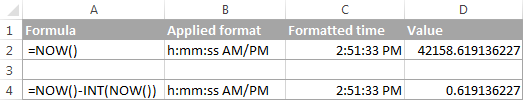### Insert time using the Excel TIME function

The TIME function in Excel is used to convert a text string showing a time into a decimal that represents the time.

The syntax of the Excel TIME function is very straightforward:

`=TIME(hour, minute, second)`

The hour, minute and second arguments can be supplied as numbers from 0 to 32767.

• If hour is greater than 23, it is divided by 24 and the remainder is taken as the hour value.

For example, TIME(30, 0, 0) equates to TIME(6,0,0), which is 0.25 or 6:00 AM.

• If minute is greater than 59, it is converted to hours and minutes. And if second is greater than 59, it is converted to hours, minutes, and seconds.

For example, TIME(0, 930, 0) is converted to TIME(15, 30, 0), which is 0.645833333 or 15:30.

The Excel TIME function is useful when it comes to merging individual values into a single time value, for example values in other cells or returned by other Excel functions.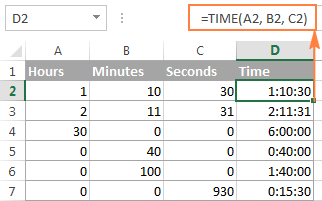## How to get hours, minutes and seconds from a timestamp

To extract time units from a time stamp, you can use the following Excel time functions:

HOUR(serial_number) - returns an hour of a time value, as an integer from 0 (12:00 am) to 23 (11:00 pm).

MINUTE(serial_number) - gets the minutes of a time value, as integers from 0 to 59.

SECOND(serial_number) - returns the seconds of a time value, as integers from 0 to 59.

In all three functions, you can input times as text strings enclosed in double quotes (for example, "6:00 AM"), as decimal numbers (e.g. 0.25 that represents 6:00 AM), or as results of other functions. A few formula examples follow below.

• `=HOUR(A2)` - returns the hours of the timestamp in cell A1.
• `=MINUTE(A2)` - returns the minutes of the timestamp in cell A1.
• `=SECOND(A2)` - returns the seconds of the timestamp in cell A1.
• `=HOUR(NOW())` - returns the current hour.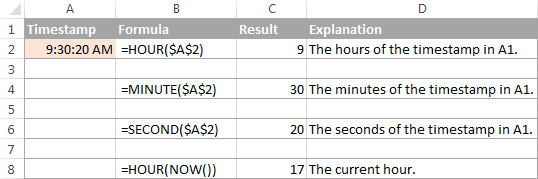Now that you've got down to brass tacks of the Excel time format and time functions, it will be much easier for you to manipulate dates and times in your worksheets. In the next part of our Excel Time tutorial, we are going to discuss different ways of converting time to numbers. I thank you for reading and hope to see you on our blog next week!